## research papersBIOLOGICALCRYSTALLOGRAPHY
ISSN: 1399-0047
Volume 64| Part 1| January 2008| Pages 11-16

## AMoRe: classical and modern

aIBS, Institut de Biologie Structurale Jean-Pierre Ebel, 41 Rue Jules Horowitz, F-38027 Grenoble; CNRS, Université Joseph Fourier; CEA, France
*Correspondence e-mail: stefano.trapani@cbs.cnrs.fr

(Received 16 February 2007; accepted 11 September 2007)

An account is given of the latest developments of the AMoRe package: new rotational search algorithms, exploitation of noncrystallographic symmetry, generation and use of ensemble models and interactive graphical molecular replacement.

### 1. Introduction

In this paper, we give an account of the latest developments of the AMoRe package. The newly introduced features follow the general guidelines that determined the success of the AMoRe molecular-replacement (MR) strategy (Navaza, 1994):

 (i) the rapid and exhaustive search for putative orientations and positions of the models by using fast' (i.e. FFT-based) search functions, which include the contribution of already fixed molecules; (ii) the subsequent assessment of the picked positional parameters based on more robust criteria (in particular, the correlation between observed and calculated structure-factor amplitudes); (iii) the possibility of assessing a large number of trial crystal configurations built up using automatic procedures for model selection and incorporation.
We describe here new rotational search algorithms (§2), the exploitation of noncrystallographic symmetry (NCS; §3), the generation and use of ensemble models (§4) and interactive graphical molecular replacement (§5).

### 2. Fast rotational sampling procedures

The rotation function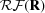, as defined by Rossmann & Blow (1962), measures the overlap between one Patterson function (the target object) and a rotated version of another (the search object) as a function of the applied rotation R. The detection of rotation-function peaks aims at determining the possible orientations of a MR probe (cross-rotation function) or the NCS rotational components (self-rotation function).

Methods for an optimal and rapid sampling of the rotation function have long been an object of study (for a review, see Navaza, 2001). Here, we describe how FFT acceleration, first introduced by Crowther (1972) to sample two-dimensional sections of the rotation domain, has been extended in AMoRe to three angular variables (Trapani & Navaza, 2006). Also, it is shown how distortion-free sections (Burdina, 1971; Lattman, 1972) can be economically sampled by FFT (Trapani et al., 2007).

A natural way of representing the rotation function is to expand it in terms of the complete set of Wigner functions, i.e. the elements of the rotation-group irreducible-representation matrices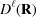(Wigner, 1959),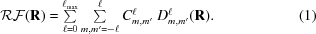This expression is easily reduced to a Fourier expansion in two-dimensional sections of the rotation domain if Euler angles (α, β, γ) are used to parametrize rotations. In fact, from the simple harmonic dependence of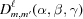on the α and γ angles,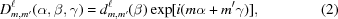it follows that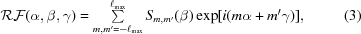where the Fourier coefficients of a β-section are given by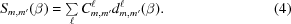(4)was first obtained by Crowther (1972) through spherical harmonic expansion of the source and target Patterson functions restricted to a spherical domain. Efficient algorithms for the evaluation of the Wigner expansion coefficients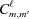were subsequently elaborated by Navaza (1993).

#### 2.1. Three-dimensional FFT sampling of the rotation function

(4)requires the evaluation of the reduced Wigner functions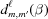up to the degree ℓmax for each sampled β value. This can be carried out by means of several recursion formulas. Alternatively, one can use the Fourier representation of,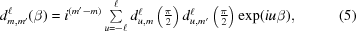which permits a full three-dimensional Fourier representation of the rotation function (Trapani & Navaza, 2006),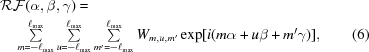where the Fourier coefficients Wm,u,m are given by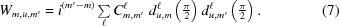Three-dimensional FFT calculation of the rotation function (6)has been implemented in AMoRe, aiming at permitting accurate and more rapid computations at high values of ℓmax for large particles such as viruses. Test calculations on the icosahedral IBDV VP2 subviral particle (ℓmax = 178) showed that the new code performs on average 1.5 times faster than the previous two-dimensional FFT-based program with no loss of accuracy. When using precalculatedcoefficients, as implemented in AMoRe, speed improvements of up to sixfold were observed.

According to Navaza (1993), calculation of theco­efficients requires the evaluation of spherical harmonic functions on a nonregular grid corresponding to the reciprocal-lattice directions. Since spherical harmonics correspond to special restrictions of the Wigner functions, (5)can be further exploited to obtain by FFT, rather than by recursion, a set of spherical harmonic values that are very finely sampled. In addition to rapidity, this approach avoids numerical stability issues found in most recursive algorithms. Accurate results up to at least ℓ = 1000 and β ≥ 10−4 rad can be obtained.

#### 2.2. Metric based FFT sampling of the rotation function

The Wigner expansion in (1)and the Fourier expansion in (6)are of quite general validity for functions defined on the domain of rotations, although they were obtained for the specific case of the Patterson-overlap rotation function. The summation limit ℓmax, which should be infinity in theory, is set to a convenient finite number in all practical cases and is generally associated with the angular resolution of the rotation function. Indeed, according to (6), ℓmax determines the maximum oscillation frequency in α, β and γ. Also, according to standard FFT requirements, ℓmax determines the minimum number (2ℓmax + 1) of equispaced samples along α, β and γ.

The choice of a suitable set of samples for the rotation function is a nontrivial issue if the actual distance between sampling points is to be taken into account. Since the metric of the rotation group, independent of its parametrization, cannot be reduced to a Euclidean metric, FFT sampling based on (6)will result in an unevenly distributed set of points in the rotation domain.

The problem can be partially solved in two-dimensional β-­sections, where the rotation metric becomes equivalent to a Euclidean metric (Burdina, 1971; Lattman, 1972). The rotation length element ds may be expressed using Euler angles as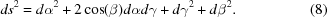It follows that in an undistorted Cartesian representation of a β-section the α and γ coordinates must define an oblique two-dimensional unit cell whose sides of length 2π form an angle equal to β (Fig. 1a). By means of an appropriate coordinate transformation, a rectangular centred unit cell can be obtained with side lengths (Fig. 1b):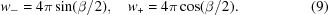A uniform sampling on these orthogonal axes will then correspond to a true constant distance Δ between rotations. Notice that the size (area) of a β-section is proportional to sinβ and that the section reduces to a one-dimensional segment if β = 0 or π.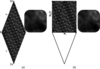Figure 1 Plot of the β = 137.8° section of the self-rotation function corresponding to the IBDV VP2 subviral particle evaluated by FFT techniques: (a) classical sampling on the primitive oblique cell; (b) metric based sampling on one independent half of the rectangular centred cell (the corresponding primitive oblique cell is outlined for reference). A detail of the two plots is also shown.

It seems physically reasonable to assume that if a sample spacing Δ (as defined above) permits the recovery of one β-­section from its samples, then the same sample spacing should also be applicable to any other β-section. Δ would then represent the angular resolution of the rotation function. Under this hypothesis, however, the number of Fourier co­efficients of a β-­section should vary according to sinβ, while, after (3)and (4), the number of Sm,m coefficients is dictated uniquely by the value of ℓmax independently of β. We have shown numerically (Trapani et al., 2007) that this apparent contradiction is resolved by an intrinsic feature of the reduced Wigner functions which renders the Sm,m coefficients vanishingly small when their indices (mm′) do not satisfy the condition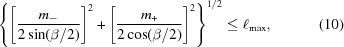with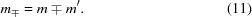(10)defines circular regions of radius lmax in the two-dimensional reciprocal sections (Fig. 2). The longest reciprocal-vector lengths to include in calculations are therefore limited by the Wigner-expansion truncation limit ℓmax. Accordingly, the resolution in direct space is given by Δ = π/ℓmax.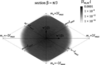Figure 2 Plot of one reciprocal β-section of the self-rotation function of the IBDV VP2 subviral particle. The {m, m′} and {m−, m+} systems of reciprocal coordinates are drawn in order to faithfully represent distances in reciprocal space.

If in (3)we limit the summation to those indices that satisfy inequality (10), then one can sample the rotation function on economic grids with sinβ fewer points than in the classical Crowther's development, while still computing it by FFT techniques, and recover distortion-free sections, which facilitates peak-searching procedures. In Fig. 1we show two plots of the same section (β = 137.8°) of the IBDV VP2 self-rotation function computed by FFT using the classical sampling (96 100 points) and the metric based sampling (64 736 points). As expected, both plots display the same features.

### 3. Exploiting NCS

When several copies of the same molecule are present in the asymmetric unit, each molecule can in principle be superimposed on another of the same type by a rigid-body movement, although the structural correspondence between the two molecules may not be exact owing to the different crystalline environments. This movement is not an element of the crystal symmetry space group; it defines a noncrystallographic symmetry operation (see Rossmann, 1990; Blow, 2001and references therein). The rotational component of the NCS operations can be detected by analysis of the self-rotation function, while no straightforward method exists for determination of the NCS translational components. An exception occurs when there is pure translational NCS, which should result in very strong peaks in the Patterson map.

The knowledge of the NCS operations can be exploited to help the MR search when the standard procedures fail.

 (i) If pure translational NCS is detected, a larger composite model formed by several copies of the search model separated by the NCS translation vectors can be used for calculation of the translation function (Navaza et al., 1998). (ii) If the NCS rotational components are determined, these can be included in the rotation search in order to enhance the signal-to-noise ratio of the rotation function (Tong, 2001). It should be noticed, however, that in some cases NCS averaging of the rotation function may just hide good orientations (Trapani et al., 2006). (iii) The translation-function steps in the standard MR procedure can be limited to only orientations of the moving molecules related to the fixed molecules by the NCS. Automatic selection/generation of NCS-related orientations is now available in the AMoRe translational procedure. (iv) The NCS operations may form an approximate symmetry point group; often this can be reliably postulated if the equivalent molecules are known to exist as an oligomeric assembly in solution. In this case, the oligomeric assembly can be constructed by using the so-called locked' search functions (Tong, 2001), which allow one to position, under the NCS restraints, several molecules at once around the centre of the assembly by varying the parameters of one molecule only. The oligomeric assembly is subsequently translated inside the unit cell. This procedure has the advantage of reducing the number of parameters to be determined and permits the use of larger models during the translation step. On the other hand, no crystal symmetry (other than the unit-cell translations) can be taken into account during the construction of the oligomeric assembly, which implies using data extended to P1. The general-purpose NCS_CORREL program calculates the locked-translation function given a set of monomer orientations and the NCS rotations. The program performs a point-by-point calculation of the correlation coefficient in terms of structure-factor amplitudes. (v) NCS may be further exploited, in the case of symmetric oligomeric proteins, when used in combination with low-resolution three-dimensional EM reconstructions of the molecules in solution (Trapani et al., 2006). The locked-translation construction of the oligomer, as described above, may be replaced by a much faster procedure in which a monomeric model, oriented according to cross-rotation function results, is fitted into an EM reconstruction (Navaza et al., 2002) of the oligomer oriented according to the NCS axis directions.

### 4. Ensemble models: taking structural variability into account

The number of protein structures available from the PDB has grown to a point where many of the known protein folds are currently over-represented. As a consequence, it is often possible to find families of homologues that are potentially exploitable as probes for a given MR problem. In these cases, the logical choice of a model must clearly favour a very closely related molecule, if one is available. On the other hand, it may be necessary to test several trial models when only medium/low-similarity homologues are available. Accumulated ex­perience shows that even if structural homology is certain, models are likely to fail if similarity is not high enough.

When the MR search using each member of a whole family of structures fails, it may be worthwhile to combine information from all available models in order to take into account structural variability within the family and thus improve the effectiveness of the model. Hybrid models can be built up on the basis of structure and sequence alignments. Their use has indeed led to positive results in some difficult MR cases. The outcome, however, depends highly on the quality of the alignment employed for model construction (Schwarzen­bacher et al., 2004). It should also be noticed that structural differences among homologues may arise not only from sequence diversity, but also from molecular flexibility, which can range from small side-chain torsional changes to large domain movements.

As an alternative to hybrid models, one can treat a whole ensemble of superposed homologous structures as an MR probe. In this way, regions of structural variability/flexibility are implicitly weighted within the model itself. This type of model closely resembles NMR-based models, whose usability as MR probes has previously been examined (Chen, 2001).

In a recent study (the results of which are briefly summarized in Table 1), we used single structures as well as ensembles of homologues to solve two difficult MR cases: the antibody Fab Q11 B13 crystal structure (unpublished data) and the Escherichia coli gene product YECD crystal structure (PDB code 1j2r ; Abergel et al., 2003). We observed that the ensembles enhanced the effectiveness of the single-structure probes. More interestingly, whole sets of individually un­fruitful structures could be correctly placed when used as ensembles. Notice that for the Fab structure rather large ensembles were used (Fig. 3). Also, many of the ensemble members corresponded to the same molecules in different crystalline environments.

 Table 1MR searches using single models and ensemble models: two test cases
 (a) Fab Q11 B13 crystal structure (unpublished data). Two-body MR search (one variable and one constant Fab domain per asymmeric unit). Resolution range: 15–3.5 Å.
CCF (%) Noise (%)
MR search using single models (173 tested, 38 successful)
Best scoring single model 39.7 30.2
MR search using ensemble models
Ensemble of 173 (all) models 42.9 34.4
Ensemble of 135 individually unsuccessful models 42.5 34.4
 (b) E. coli gene product YECD (PDB code 1j2r ). Four-body MR search (one tetrameric assembly per aymmetric unit). Resolution range: 15–3.5 Å.
CCF (%) Noise (%)
MR search using (monomeric) single models (15 tested, 3 successful)
Best scoring single model 20.2 16.1
MR search using (monomeric) ensemble models
Ensemble of 15 (all) models 29.8 23.0
Ensemble of 12 individually unsuccessful models 28.7 22.2
 (c) Electron-density correlation (EDC) of the least and most similar pairs of structures in the ensembles.
Resolution (Å) Worst EDC (%) Best EDC (%)
Fab constant domains 15–4.0 44.5 92.5
Fab variable domains 15–4.0 33.7 88.2
E. coli YECD homologues 15–3.5 36.8 94.4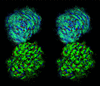Figure 3 Stereo image of the ensembles of Fab constant-domain and variable-domain structures.

In order to superpose molecular structures and thus generate ensembles, it is common practice to use algorithms which optimize a certain set of interatomic distances. In the work described above, we used a different approach based on the maximization of the electron-density correlation (EDC). By expressing the EDC in terms of the molecular Fourier transforms, the superposition problem can be straight­forwardly reduced to an MR-like problem. Exploiting the existing AMoRe procedures, an automatic EDC-based model-superposition utility, SUPER, has been developed and is now available as part of the software package. A somewhat related technique has been implemented in MOLREP (Vagin & Isupov, 2001). Although both approaches aim to maximize the overlap between two electron densities considered as rigid bodies, in MOLREP the putative translations are first determined by means of the spherically averaged phased translation function and the orientations are then looked for by means of a phased rotation function, whereas in SUPER we first use the standard fast rotation function to determine the putative orientations and then compute the phased translation function. The EDC maximization presents some advantages with respect to distance-based superposition methods.

 (i) EDC is a convenient measure of structural similarity between models in an MR context, for the MR method can ultimately be thought of as a matching procedure between the model and the crystal electron density. (ii) EDC is more meaningful than sequence similarity, which does not consider molecular flexibility, or atomic position r.m.s.d., which implies the existence of `equivalent' atoms. (iii) When the EDC is calculated in reciprocal space, structure similarity can be evaluated at different resolution levels. Also, model superposition can be carried out at any desired resolution. (iv) Remote homologues (and, in principle, even unrelated structures) can be superposed without requiring the definition of any equivalence between atoms or residues (no need for sequence alignments). (v) No modelling of variable regions is needed.

According to our experience, ensemble-based MR searches have the potential to combine and exploit the richness of structural information in the PDB in a relatively easy though effective way. EDC-based ensembles of structures should be considered by developers of databases for automatic structure-solution pipelines as a valuable alternative to homology-based representative models.

### 5. Graphical interactive molecular replacement

A molecular-graphics interface has been developed to assist the AMoRe user in the interpretation and interactive manipulation of the MR search results. The program permits the following.

 (i) Direct loading and display of the molecular arrangements corresponding to the positional parameters listed in the AMoRe output files, with no need to generate the corresponding PDB coordinate files. (ii) Easy access to the crystal packing by displaying the content of several adjacent unit cells. The user has the option to switch on or off the visibility of any molecule according to several selection criteria; in addition, an arbitrary portion of the crystal can be clipped using a movable clipping box of variable size. (iii) Translate/rotation of any displayed molecule using click-and-drag mouse mechanisms; all symmetry-related mates follow, in real time, the displacements of the moving molecule. (iv) The calculation in real time, while the user moves a molecule, of the correlation between calculated and experimental structure-factor amplitudes of the crystal. Optionally, the correlation of structure-factor intensities and the R factor are also available. Calculations are carried out in a user-defined resolution range. A correlation bar is displayed on the screen to visually help the user to follow the changes in the correlation values. (v) The performance of rigid-body refinement (through calls to the AMoRe FITING program) of the molecular positional parameters starting from the displayed crystal configuration.

A foreseen use of the program, in addition to facilitating crystal-packing analysis, is for the specific cases in which one wants to position small-size components of molecular complexes, especially when there is some prior knowledge of the regions of interaction between the components.

### Acknowledgements

We acknowledge Alberto Podjarny for kindly providing the Fab Q11 B13 crystal structure factors.

### ReferencesBIOLOGICALCRYSTALLOGRAPHY